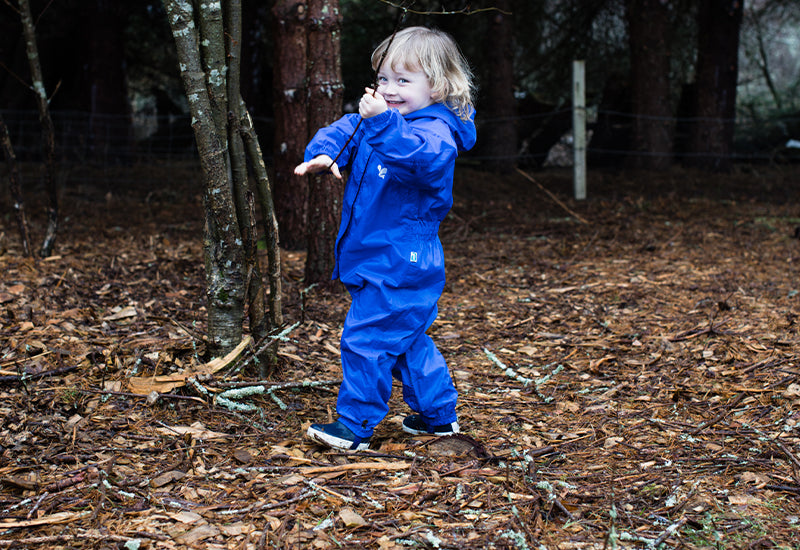# Learning Objectives

To recall and use multiplication facts for the 2, 5 and 10 multiplication tables, including recognising odd and even numbers

To add and subtract numbers using concrete objects

- Elastic bands

- Sticks

### Introduction

Children to sit in a circle to recite 2, 5 and 10 multiplication tables

Ask children to collect as many sticks as they can

### Activity

Ask children to group the sticks in groups of 2, 5 and 10 depending on the level of the group

Children to put elastic bands around their groups of sticks

Children to then place stick groups in front of them, and recite the appropriate times table using the sticks as visual aids

Children can also be given addition and subtraction problems, and then use the sticks to solve the problems.